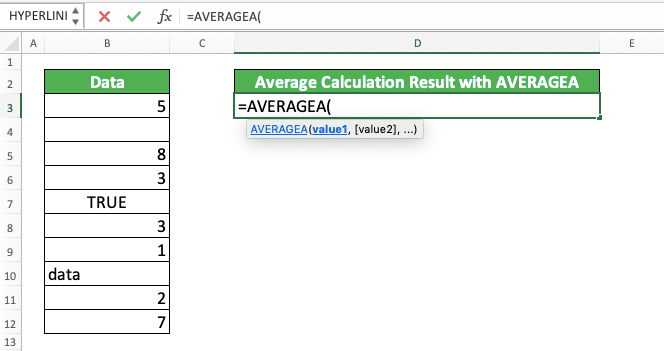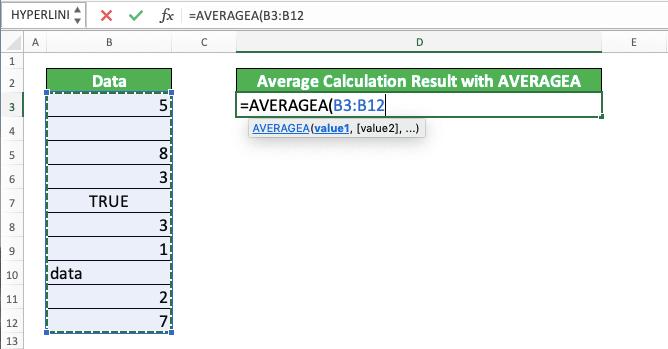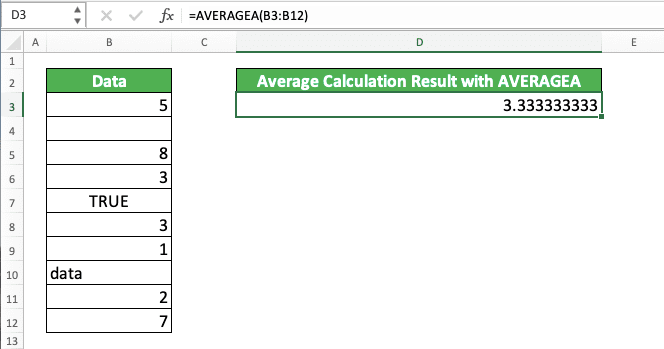AVERAGEA Formula in Excel: Functions, Examples, and How to Use - Compute Expert

# AVERAGEA Formula in Excel: Functions, Examples, and How to Use

Home >> Excel Tutorials from Compute Expert >> Excel Formulas List >> AVERAGEA Formula in Excel: Functions, Examples, and How to Use

In this tutorial, you will learn completely about AVERAGEA in excel.

As a formula, AVERAGEA can average the data you have just like AVERAGE, a more popular formula in excel. However, AVERAGEA has some uniqueness that makes it an intriguing formula to use in special circumstances.

Curious to know more about AVERAGEA? Read this tutorial until the end!

Disclaimer: This post may contain affiliate links from which we earn commission from qualifying purchases/actions at no additional cost for you. Learn more

## What is AVERAGEA in Excel?

AVERAGEA is a formula that can help you to average your data in excel. AVERAGEA involves logic values and text in its calculation process.

## AVERAGEA Function in Excel

AVERAGEA can make us know the average of our data which includes numbers, text, and logic values.

## AVERAGEA Result

The result we get from AVERAGEA is a number that represents our data average.

## Excel Version from Which We Can Use AVERAGEA

We can start using AVERAGEA since excel 2003.

## The Way to Write It and Its Inputs

Here is a general writing form of AVERAGEA in excel.

= AVERAGEA ( value1 , [ value2 ] , … )

The inputs there are the values you want to calculate the average of with AVERAGEA. They can be numbers, text, or logic values.

AVERAGEA assumes a TRUE logic value as 1, while text and a FALSE logic value are assumed as 0.

We can input these values in the forms of direct values, cell coordinates, or cell ranges. If we give more than one inputs, then we need to separate them using comma symbols ( , ).

We usually just input one cell range, which contains all the values we want to average, to AVERAGEA. In its processing, AVERAGEA will ignore blank cells that we input into it.

## Example of Its Usage and Result

You can see the example of AVERAGEA implementation and result below.In the example, we use AVERAGEA to calculate the average of data in our column.

The way to write the AVERAGEA formula is quite easy as you can see above. We just need to input the cell range where all the data we want to average are in.

For the example’s data processing, AVERAGEA assumes the “Data” text there as 0 and TRUE as 1. It also ignores the blank cell in the column cell range.

This means AVERAGEA calculates the average of 5, 8, 3, 1, 3, 1, 0, 2, and 7 in the example. The result for this is 3.333333333!

## Writing Steps

In this part, we will discuss the detailed steps to write an AVERAGEA in excel. If you have some troubles when you try to use the formula, just follow these writing steps!

1. Type an equal symbol ( = ) in the cell where you want to put your AVERAGEA result2. Type AVERAGEA (can be with small or capital letters) and an open bracket symbol3. Input all the values you want to average by using direct typing/cell coordinates/cell ranges. Separate all of your inputs with comma symbols4. Type a close bracket symbol after you have inputted all the values you want to average5. Press Enter
6. Done!## Difference Between AVERAGE and AVERAGEA

What are the differences between these two almost similar formulas, AVERAGE and AVERAGEA?

If you have understood AVERAGE before and have followed the tutorial until this point, you may have noticed some differences. However, to make it simpler for you, here is a table that summarizes them.

AVERAGEAVERAGEA
Ignore textInvolve text. Assumes them as 0
Ignore logic valuesInvolve logic values. Assumes TRUE as 1 and FALSE as 0
Ignore empty quotes ( “” )Involve empty quotes. Assumes them as 0

Both AVERAGE and AVERAGEA ignore empty cells and will produce an error if there is an error in their inputs.

To give you a clearer understanding of the AVERAGE and AVERAGEA comparison, here is an example to illustrate it.We use some sets of data there to give a clear picture of the AVERAGE and AVERAGEA comparison.

As you can see, AVERAGE and AVERAGEA differ in results when the data they average involves logic values and text. AVERAGE ignores them while AVERAGEA involves them with its assumptions.

When there aren’t logic values, text, or empty quotes, AVERAGE and AVERAGEA produce the same results. This includes when there is an error in the values they average (both will result in an error because of this)!

## Exercise

After we have discussed completely about AVERAGEA in excel, now let’s do an exercise. This is so you can deepen your understanding of how to use the formula!

### Questions

Answer all these questions using AVERAGEA!
1. Average all the data in the “Data” column!
2. Average all the data in the “Data” column! Include one TRUE and one FALSE in the average calculation!
3. Average all the data in the “Data” column! Include the column header and cell B15 in the average calculation!

• If you want to input text to AVERAGEA, you must input it using a cell coordinate/cell range
• You can give up to 255 different inputs in AVERAGEA

Other tutorials you should learn:

Get updated excel info from Compute Expert by registering your email. It's free!